### Navier-Stokes Equations

In each phase of the two-phase problem the flow is described by the instationary Navier-Stokes equations: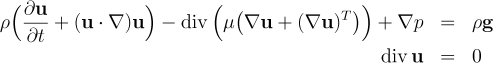The density and the viscosity are constant, but differ for the two phases. In addition suitable initial and boundary conditions are needed.

Notation: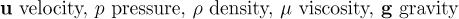### Continuum Surface Force

A standard assumption is that the surface tension balances the stress at the phase-interface. This can be modeled by a force localized at the interface, using the so-called continuum surface force (CSF) technique: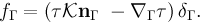This term is added as a force term in the momentum equation of the Navier-Stokes equations.

Notation: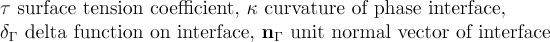### Mass Transport

The concentration of a dissolved species in one or both phases, that is transported due to convection and diffusion and does not adhere to the interface, can be modeled by the convection-diffusion equation for the concentration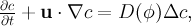At the interface we need interface conditions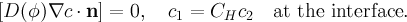Notation: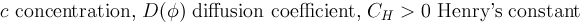### Surfactant

The concentration of a surfactant at the interface, can be modeled by the transport equation for the concentration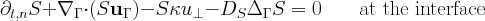Notation: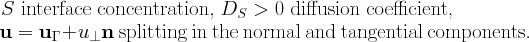### Level Set

The interface between the two phases is captured by a so-called level set function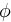: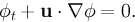This continuous scalar function is positive in one phase and negative in the other. The interface is the 0-level of the function - hence the name. The level set function, and therefore the interface, is advected by the flow field.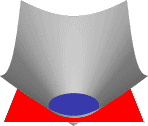### Fast Marching

 For numerical and algorithmic purposes it is necessary to keep the level set function close to a signed distance function during the time evolution. We accomplish this by a reinitialization of the level set function using a variant of the Fast Marching method.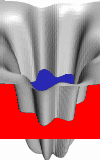The spatial discretization is based on a hierarchy of tetrahedral grids. The grids are consistent, i.e. there are no hanging nodes. An important property is that local refinement and coarsening are easy to realize. The local refinement is crucial for an accurate and efficient approximation of the interface. The hierarchical structure is exploited by the multi-level solvers.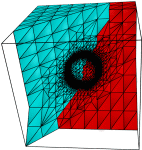### Refinement Algorithm

 To ensure consistency of the grids, the refinement pattern of adjacent tetrahedra has to match on common faces and edges. For this purpose DROPS provides a regular refinement pattern (with all six edges refined) for a tetrahedron and 63 irregular patterns for each possible pattern on the edges. The patterns are chosen such that matching patterns on the edges imply matching patterns on the faces. The rule that no irregular pattern can be refined (but has to be replaced by the regular pattern that can be refined instead) guarantees that the hierarchy of triangulations is stable. That means that the tetrahedra will not degenerate, i.e. all angles are uniformly bounded away from 0° and 180°.### Finite Elements

 For the discretization of velocity, pressure and the level set function conforming finite elements are used. The Hood-Taylor P2-P1 finite element pair is used for velocity / pressure and the P2 finite element is used for the level set function. We introduced an extended finite element (XFE) space for the pressure which is suited to represent functions which are discontinuous across the interface. For such functions this XFE method has a better approximation property of order 2 instead of order 1/2 for standard finite element spaces.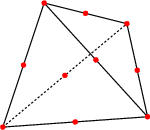### Curvature Approximation via Laplace-Beltrami

 The CSF term contains two parts that should not be handled naively: the delta function and the curvature (which is essentially a second derivative). To eliminate the delta function, the integral of the weak formulation is written as surface integral over the phase interface. The second derivative introduced by the curvature can be interpreted as a Laplace-Beltrami operator. In the weak formulation the second derivative is transferred to the test function by a variant of partial integration for surfaces. To approximate the resulting surface integrals, which only contain first derivatives, a piecewise linear reconstruction of the 0-level of the piecewise quadratic level set function on a finer grid is used.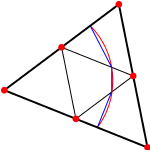### Nested Solvers

The instationary, non-linear and strongly coupled nature of the mathematical problem is mirrored in the structure of the solvers:

 For the time integration we use a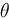-scheme. The Navier-Stokes equations and the advection equation for the level set function are decoupled via a fixed point iteration approach which iterates between the advection equation and the Navier-Stokes equations. A fixed point iteration is also used to tackle the non-linear convection term in the Navier-Stokes equations: In each iteration the equations are linearized using the result of the previous one. The remaining Oseen problem can be handled by inexact Uzawa or preconditioned GCR solvers. These solvers require inner solvers (preconditioners) for scalar elliptic problems, for example, a Poisson equation or a convection diffusion equation. DROPS offers preconditioned conjugate gradient(PCG), Krylov subspace methods (GMRES, BiCGSTAB, GCR) and multigrid (MG) methods for this task. As preconditioners / smoothers Jacobi or Gauss-Seidel methods can be used. Coupled multigrid methods with Braess-Sarazin or Vanka smoothers are also used to solve the generalized Stokes problem.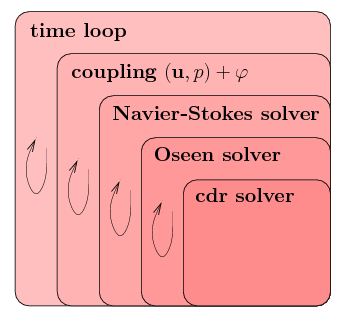### Software Design

DROPS is written in C++, making heavy use of the object-oriented features of the programming language. This holds especially for the nested solvers with its preconditioners, smoothers etc. In order not to sacrifice speed only compile-time polymorphism is used.

### Data Structures

In DROPS there's a strict separation between the data structures for the geometry related data and the linear algebra related ones. This simplifies the algorithms (in particular solvers) and allows for high performance (lean data structures for positive cache effects which are optimized for fast matrix-vector multiplication).

DROPS has special data structures for all types of simplices in the grid, i.e. vertices, edges, faces, and tetrahedra. Nodes of different types of finite elements can therefore easily be associated with the respective simplices.

Vectors are based on the `valarray` class in C++. This allows DROPS to use simple notations while not sacrificing performance due to the implementation of valarray via expression templates. For the sparse matrices DROPS uses a compact row-oriented storage format that only stores the non-zero entries. During assembly of these matrices an intermediate storage format is used as insertion of entries into the final format is not cheap.

The vectors and matrices are connected to the geometric structure via index classes. These are used for example during assembly of the linear systems. Other important classes for the assembly are classes that represent single finite elements and classes that perform quadrature on them.

### Grid Generation and Visualization

For the generation of the initial grid DROPS relies on external software. The data format used by the GAMBIT grid generator can be imported.

DROPS supports several software packages for visualization: Ensight, Tecplot, and Geomview. For further data processing ASCII output is available.

### Overall Structure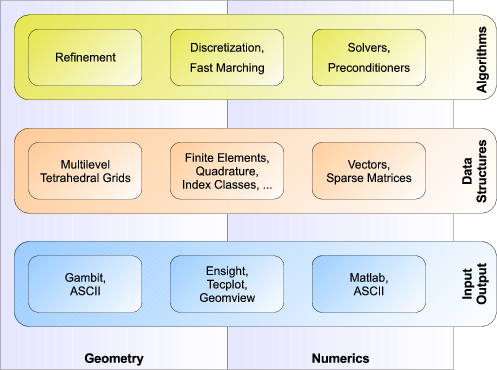Sven Groß ✉, last update: February 24th, 2008